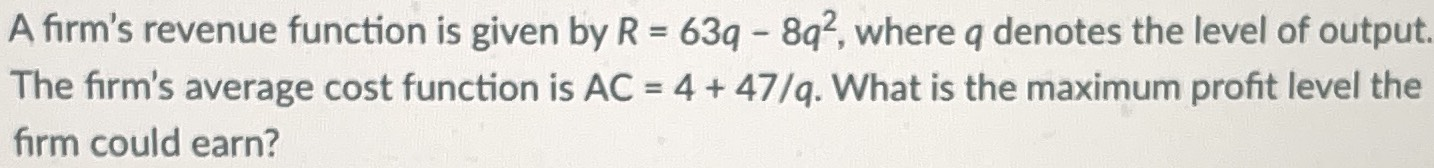### ¿Todavía tienes preguntas de matemáticas?

Pregunte a nuestros tutores expertos
Algebra
PreguntaA firm's revenue function is given by $$R = 63 q - 8 q ^ { 2 }$$ , where $$q$$ denotes the level of output. The firm's average cost function is $$A C = 4 + 47 / q$$ . What is the maximum profit level the firm could earn?